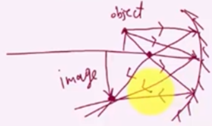Need Help?

Subscribe to Physics 2

###### \${selected_topic_name}
• Notes

$\begin{array}{l}{\text { An object } 0.600 \mathrm{cm} \text { tall is placed } 16.5 \mathrm{cm} \text { to the left of the }} \\ {\text { vertex of a concave spherical mirror having a radius of curvature }} \\ {\text { of } 22.0 \mathrm{cm} \text { . (a) Draw a principal-ray diagram showing the forma- }} \\ {\text { tion of the image. (b) Determine the position, size, orientation, and }} \\ {\text { nature (real or virtual) of the image. }}\end{array}$

$y=0.6 \mathrm{cm}$

$S=16.5 \mathrm{cm}$

$R=22 cm$

$\frac{1}{s}+\frac{1}{s'}=\frac{2}{R} \Rightarrow ∴ \frac{1}{16 \cdot 5}+\frac{1}{s'}=\frac{2}{22}$

$∴ \frac{1}{s^{\prime}}=\frac{2}{22}-\frac{1}{16 \cdot 5} \Rightarrow S'= 33 cm>0$

$M=\frac{-S^{\prime}}{S}$$\Rightarrow m=\frac{-33}{16.5}$$=-2(m<0,|m|>1)$

$\left|y^{\prime}\right|=|m \| y|$

$\left|y^{\prime}\right|=2 * 0.6=1.2 cm$$\begin{array}{l}{\text { You hold a spherical salad bowl } 90 \mathrm{cm} \text { in front of your }} \\ {\text { face with the bottom of the bowl facing you. The salad bowl is }} \\ {\text { made of polished metal with a } 35-\mathrm{cm} \text { radius of curvature. (a) }} \\ {\text { Where is the image of your 2.0-cm-tall nose located? (b) What are }} \\ {\text { the image's size, orientation, and nature (real or virtual)? }}\end{array}$

$S=90 \mathrm{cm}$

$R=35 \mathrm{cm}$

$y=2 \mathrm{cm}$

$∵ \frac{1}{s}+\frac{1}{s'}= \frac{2}{R} \Rightarrow \frac{1}{90}+\frac{1}{s'}=\frac{2}{-35}$

$∴ =\frac{1}{s'}=\frac{-2}{35}-\frac{1}{90} \Rightarrow {s'}\simeq -14.65 cm<0$

$∵ m=\frac{-s^{\prime}}{s}= \frac{-(-14 \cdot 65)}{90} \simeq(0.163)>0$

$|m|<|$

$\left|y^{\prime}\right|=|m||y|$$\Rightarrow y^{\prime}=0.163*2\simeq0.326 \simeq 0.33 cm$